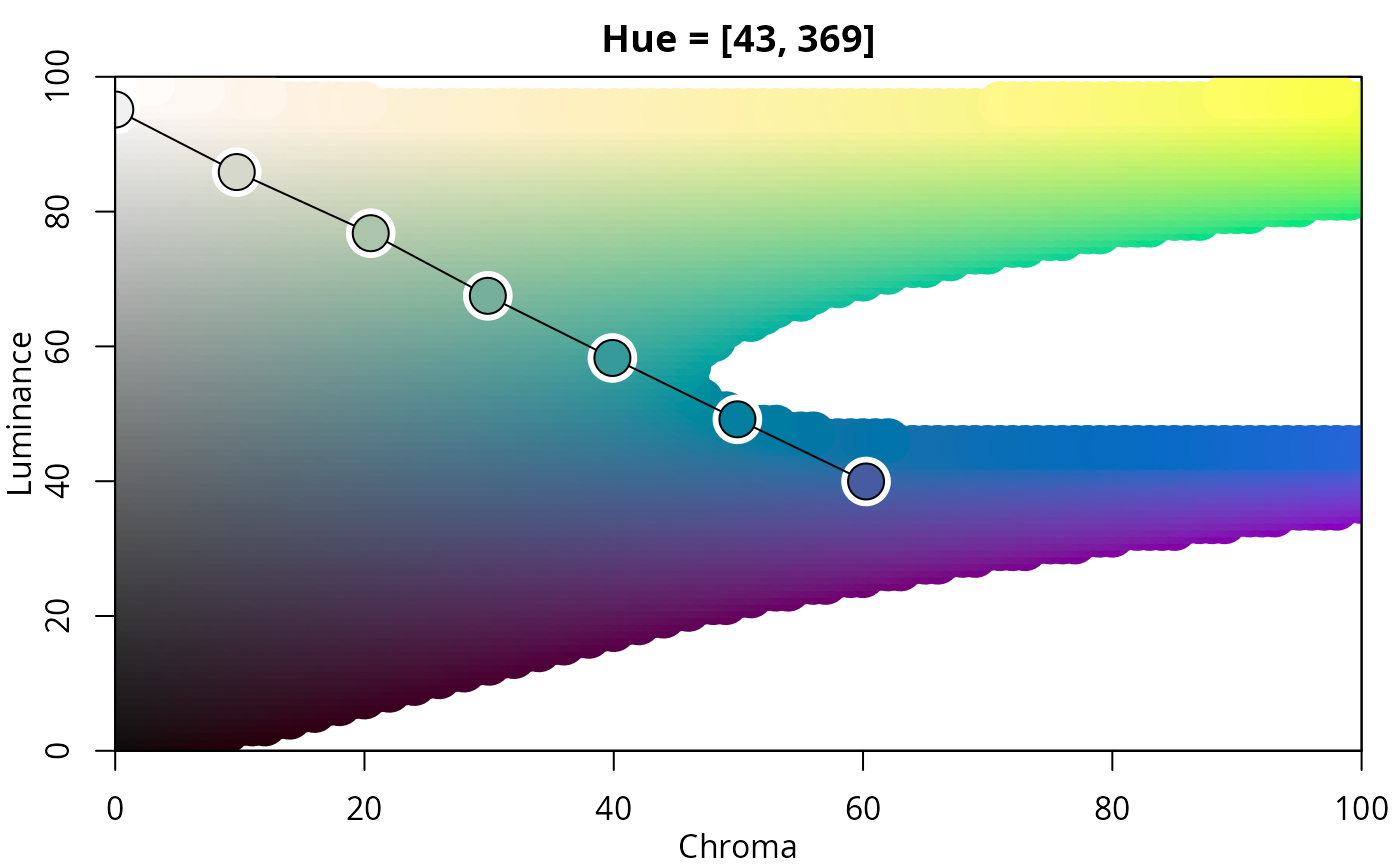Visualization of color palettes in HCL space projections.

hclplot(
x,
type = NULL,
h = NULL,
c = NULL,
l = NULL,
xlab = NULL,
ylab = NULL,
main = NULL,
cex = 1,
axes = TRUE,
bg = "white",
lwd = 1,
size = 2.5,
...
)

## Arguments

x

character vector containing color hex codes, or a color-class object.

type

type character specifying which type of palette should be visualized ("qualitative", "sequential", or "diverging"). For qualitative palettes a hue-chroma plane is used, otherwise a chroma-luminance plane. By default, the type is inferred from the luminance trajectory corresponding to x.

h

numeric hue(s) to be used for type = "sequential" and type = "diverging". By default, these are inferred from the colors in x.

c

numeric. Maximal chroma value to be used.

l

numeric luminance(s) to be used for type = "qualitative". By default, this is inferred from the colors in x.

xlab, ylab, main

character strings for annotation, by default generated from the type of color palette visualized.

cex

numeric character extension.

axes

logical. Should axes be drawn?

bg, lwd, size

graphical control parameters for the color palette trajectory.

...

currently not used.

## Value

hclplot invisibly returns a matrix with the HCL coordinates corresponding to x.

## Details

The function hclplot is an auxiliary function for illustrating the trajectories of color palettes in two-dimensional HCL space projections. It collapses over one of the three coordinates (either the hue H or the luminance L) and displays a heatmap of colors combining the remaining two dimensions. The coordinates for the given color palette are highlighted to bring out its trajectory.

The function hclplot has been designed to work well with the hcl_palettes in this package. While it is possible to apply it to other color palettes as well, the results might look weird or confusing if these palettes are constructed very differently (e.g., as in the highly saturated base R palettes).

More specifically, the following palettes can be visualized well:

• Qualitative with (approximately) constant luminance. In this case, hclplot shows a hue-chroma plane (in polar coordinates), keeping luminance at a fixed level (by default displayed in the main title of the plot). If the luminance is, in fact, not approximately constant, the luminance varies along with hue and chroma, using a simple linear function (fitted by least squares). hclplot shows a chroma-luminance plane, keeping hue at a fixed level (by default displayed in the main title of the plot). If the hue is, in fact, not approximately constant, the hue varies along with chroma and luminance, using a simple linear function (fitted by least squares.

• Diverging with two (approximately) constant hues: This case is visualized with two back-to-back sequential displays.

To infer the type of display to use, by default, the following heuristic is used: If luminance is not approximately constant (range > 10) and follows rougly a triangular pattern, a diverging display is used. If luminance is not constant and follows roughly a linear pattern, a sequential display is used. Otherwise a qualitative display is used.

specplot

## Examples

## for qualitative palettes luminance and chroma are fixed, varying only hue
hclplot(qualitative_hcl(9, c = 50, l = 70))## single-hue sequential palette (h = 260) with linear vs. power-transformed trajectory
hclplot(sequential_hcl(7, h = 260, c = 80, l = c(35, 95), power = 1))hclplot(sequential_hcl(7, h = 260, c = 80, l = c(35, 95), power = 1.5))## advanced single-hue sequential palette with triangular chroma trajectory
## (piecewise linear vs. power-transformed)
hclplot(sequential_hcl(7, h = 245, c = c(40, 75, 0), l = c(30, 95), power = 1))hclplot(sequential_hcl(7, h = 245, c = c(40, 75, 0), l = c(30, 95), power = c(0.8, 1.4)))## multi-hue sequential palette with small hue range and triangular chroma vs.
## large hue range and linear chroma trajectory
hclplot(sequential_hcl(7, h = c(260, 220), c = c(50, 75, 0), l = c(30, 95), power = 1))hclplot(sequential_hcl(7, h = c(260, 60), c = 60, l = c(40, 95), power = 1))## balanced diverging palette constructed from two simple single-hue sequential
## palettes (for hues 260/blue and 0/red)
hclplot(diverging_hcl(7, h = c(260, 0), c = 80, l = c(35, 95), power = 1))